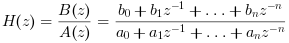Scilab Home page | Wiki | Bug tracker | Forge | Mailing list archives | ATOMS | File exchange
Change language to: English - Français - Português - 日本語 -
Справка Scilab >> Signal Processing > Filters > filter

filter

filters a data sequence using a digital filter

Syntax

[y,zf] = filter(B, A, x [,zi])

Arguments

B

real vector : the coefficients of the filter numerator in decreasing power order, or a polynomial.

A

real vector : the coefficients of the filter denominator in decreasing power order, or a polynomial.

x

real row vector : the input signal

zi

real row vector of length max(length(a),length(b))-1: the initial condition relative to a "direct form II transposed" state space representation. The default value is a vector filled with zeros.

y

real row vector : the filtered signal.

zf

real row vector : the final state. It can be used to filter a next batch of the input signal.

Description

This function filters a data sequence using a digital filter using a "direct form II transposed" implementation.

The filter canonical form is :The algorithm uses the highest degree between degree(a) and degree(b) as value for n.

If the polynomial form is used for B (resp. for A) then a polynomial or a scalar must be used for A (resp. B).

References

Oppenheim, A. V. and R.W. Schafer. Discrete-Time Signal Processing, Englewood Cliffs, NJ: Prentice-Hall, 1989, pp. 311-312.

Examples

x = [1 zeros(1,9)]
h = [0 0 1];
res = filter(h, 1, x) //This creates a delay of 2 elements

z = poly(0, "z");
B = 1;
A = z^2;
// B/A is z^(-2)
// the resulting filter is also a delay of 2 elements
res = filter(B, A, x)

//Integrator filter
x = ones(1,10)
B = 1;
A = [1 -1];
res = filter(B, A, x)

• flts — time response (discrete time, sampled system)
• rtitr — discrete time response (transfer matrix)
• ltitr — discrete time response (state space)